# Electric field

In physics, an electric field consists of vectors that act on electric charges. The force on a charge has a strength equal to the intensity of the field times the magnitude of the charge. The force on the charge is in the direction of the electric vector when the electric charge is positive and in opposite direction when the charge is negative. The electric field may vary in intensity and direction from point to point in space, it is then called inhomogeneous. The field may vary in time, in which case it is accompanied by a time-dependent magnetic field; the two time-dependent fields together form an electromagnetic field.

An electric field has dimension force per unit of charge or, equivalently, voltage per length. In the SI system, the appropriate units are newton per coulomb, equivalent to volt per meter. In Gaussian units, the electric field is expressed in units of dyne per statcoulomb (formerly known as esu), equivalent to statvolt per centimeter.

## Definition

Because an electric field E is a vector field, it is defined at every point r of space. Usually the space considered here is the non-relativistic 3-dimensional space—the Euclidean 3-space. A charge q at r experiences the force: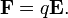$\mathbf{F} = q \mathbf{E}.$

Often E is caused by the presence of one or more electric charges other than q, but it may also be caused by a magnetic field that varies in time, or by a combination of the two. The expression states that the direction of E is such that a positive charge q is pushed in the direction of E (F and E parallel), while for a negative charge F and E are anti-parallel.

The length |E| of E at a certain point is the strength of the electric field in that point, also known as the field intensity in that point. The strength |E| ≡ E may be defined as the magnitude F ≡ |F| of the electric force exerted on a unit positive electric test charge q, or for arbitrary q by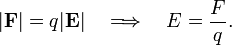$|\mathbf{F}| = q |\mathbf{E}|\quad\Longrightarrow\quad E = \frac{F}{q}.$

The strength of the electric field does not depend on the test charge q. Strictly speaking, the introduction of a small test charge, which itself causes an electric field, slightly modifies the existing field. The electric field may therefore be defined as the force per positive charge Δq that is so small that the field can be assumed undisturbed by the presence of Δq. The strength of the electric field due to a single point charge is given by Coulomb's law.

An electric field may be time-dependent, as in the case of a field caused by charges going back and forth in the antenna of a television station. Such a field is always accompanied by a magnetic field. The electric field with an accompanying magnetic field is propagated through space as an electromagnetic wave at the same speed as that of light.

When there is no time-dependent magnetic field present, the electric field E is related to an electric potential Φ,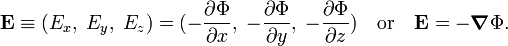$\mathbf{E} \equiv (E_x,\; E_y,\; E_z) = (-\frac{\partial \Phi}{\partial x},\; -\frac{\partial \Phi}{\partial y},\;-\frac{\partial \Phi}{\partial z})\quad\hbox{or}\quad \mathbf{E} = -\boldsymbol{\nabla} \Phi.$

## Mathematical description

An electric field E may be due to the presence of charges by Gauss's law, which in differential form is one of the microscopic Maxwell equations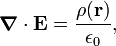$\boldsymbol{\nabla} \cdot \mathbf{E} = \frac{\rho(\mathbf{r})}{\epsilon_0},$

where ε0 is the electric constant, ρ(r) is a charge distribution, and · stands for the divergence of E.

The field may also be caused by a varying magnetic field as shown by Faraday's law (one of Maxwell's equations),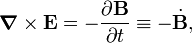$\boldsymbol{\nabla} \times \mathbf{E}= -\frac{\partial \mathbf{B}}{\partial t}\equiv - \dot{\mathbf{B}},$

where B is the magnetic flux density (also known as magnetic induction), and the symbol × stands for the curl of E.

Because of the Helmholtz decomposition of a general vector field, we can write$\mathbf{E} = \boldsymbol{\nabla}\times \mathbf{C}(\mathbf{r}) -\boldsymbol{\nabla}\Phi(\mathbf{r})$

with$\Phi(\mathbf{r}) = \frac{1}{4\pi \epsilon_0} \int \frac{\rho(\mathbf{r}')}{|\mathbf{r}-\mathbf{r}'|} d^3\mathbf{r}',$

which is the instantaneous (non-retarded) Coulomb potential due to ρ(r), and$\mathbf{C}(\mathbf{r}) = - \frac{1}{4\pi } \int \frac{\dot{\mathbf{B}}} {|\mathbf{r}-\mathbf{r}'|} d^3\mathbf{r}' .$

The field C(r) is related to the time derivative of the vector potential A if we require the Coulomb gauge. We introduce A and the Coulomb gauge, respectively:$\mathbf{B} = \boldsymbol{\nabla}\times\mathbf{A}, \qquad \boldsymbol{\nabla}\cdot\mathbf{A} = 0.$

One can then show that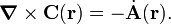$\boldsymbol{\nabla}\times\mathbf{C}(\mathbf{r}) =- \dot{\mathbf{A}}(\mathbf{r}).$

Hence the Helmholtz decomposition of the electric field (together with Coulomb gauge) gives the general expression for the electric field (in SI units):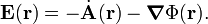$\mathbf{E}(\mathbf{r}) = -\dot{\mathbf{A}}(\mathbf{r}) -\boldsymbol{\nabla}\Phi(\mathbf{r}).$

In Gaussian units this equation reads:$\mathbf{E}(\mathbf{r}) = -\frac{1}{c}\dot{\mathbf{A}}(\mathbf{r}) -\boldsymbol{\nabla}\Phi(\mathbf{r}),$

where c is the speed of light in vacuo. Clearly, when the time derivative of A vanishes, the field E is minus the gradient of the electric potential Φ.

### Special relativity

In a relativistic formulation one defines the covariant electromagnetic four-potential φ as,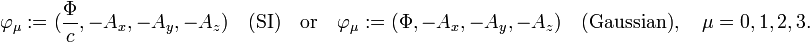$\varphi_\mu := (\frac{\Phi}{c}, -A_x, -A_y, -A_z) \quad\hbox{(SI)}\quad\hbox{or}\quad \varphi_\mu := (\Phi, -A_x, -A_y, -A_z) \quad\hbox{(Gaussian)},\quad \mu=0,1,2,3.$

where the Minkowksi metric has signature (+, −, −, −). The four-gradient is: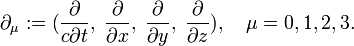$\partial_\mu := (\frac{\partial}{c\partial t},\; \frac{\partial}{\partial x},\; \frac{\partial}{\partial y},\; \frac{\partial}{\partial z}),\quad \mu=0,1,2,3.$

Hence (SI):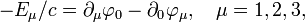$- E_\mu/c = \partial_\mu \varphi_0 - \partial_0 \varphi_\mu, \quad \mu=1,2,3,$

and (Gaussian):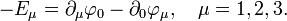$-E_\mu = \partial_\mu \varphi_0 - \partial_0 \varphi_\mu, \quad \mu=1,2,3.$

Minus the electric field (augmented with E0 = 0 and divided by c in the case of SI units) is the first (label 0) column of the (antisymmetric) electromagnetic field tensor,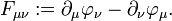$F_{\mu\nu} := \partial_\mu\varphi_\nu - \partial_\nu\varphi_\mu.$